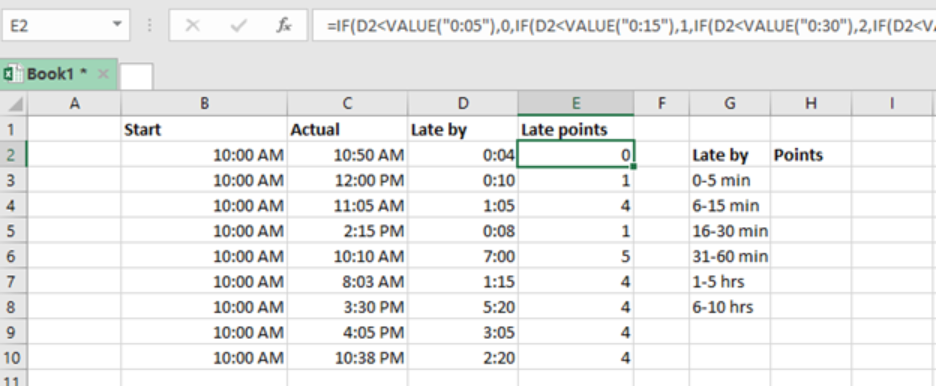Get instant live expert help with Excel or Google Sheets“My Excelchat expert helped me in less than 20 minutes, saving me what would have been 5 hours of work!”

#### Post your problem and you’ll get Expert help in seconds.

Your message must be at least 40 characters
Our professional Expert are available now. Your privacy is guaranteed.

# Assign points based on late time

There are times when we need to assign penalty points on late time. To do this, we can utilize a nested IF formula. This post provides a clear guide on how to use this function to assign points on late time in excel.Figure 1: How to assign points on late time

## General syntax of the formula

`=IF(time<VALUE(“0:05”),0,IF(time<VALUE(“0:15”),1,IF(time)<VALUE(“0:30”),2,IF(time)<VALUE(“0:60”),3,IF(time)<VALUE(“6:00”),4,5)))))`

Where;

Time- is the “late by” time

## How the formula works

This formula tests the threshold values in an ascending order. The formula first checks the “late by” column, which is column D2:D10 in the above example, before returning an answer for the late points.

In our case above, the formula for cell E2 is

`  =IF(D2<VALUE(“0:05”),0,`

• If the condition is satisfied, that is if it is less than 0:05, then it will assign it zero points.
• In the event that the logical test turns out to be FALSE, the formula checks D2 is less than the next threshold, which is 0:15, then it will assign it 1 points.
• This pattern keeps repeating itself for each threshold.
• We use the VALUE function in the formula to enable it treat the threshold as a number and not as text.

Most of the time, the problem you will need to solve will be more complex than a simple application of a formula or function. If you want to save hours of research and frustration, try our live Excelchat service! Our Excel Experts are available 24/7 to answer any Excel question you may have. We guarantee a connection within 30 seconds and a customized solution within 20 minutes.

### Did this post not answer your question? Get a solution from connecting with the expert.Another blog reader asked this question today on Excelchat:
Solution examplesGiven: RetYr = 2057 B48=2055 B49=2056 B50=2057 FirstYrAnnExp = 174,855 B51=2058 M48=IF(B48>=RetYr-1,IF(M47>1,M47*(1+Inflation),FirstYrAnnExp)) Returns FALSE M49=IF(B49>=RetYr-1,IF(M48>1,M48*(1+Inflation),FirstYrAnnExp)) Correctly retuns 0 M50=IF(B50>=RetYr-1,IF(M49>1,M49*(1+Inflation),FirstYrAnnExp)) Correctly returns 174,855 M51=IF(B51>=RetYr-1,IF(M50>1,M50*(1+Inflation),FirstYrAnnExp)) Correctly returns 180,100 How can I avoid â€œFALSEâ€ when the B column is less than RetYr? A zero or blank would be better.
Solved by V. W. in 20 minsHi Can you see any glaring error with the below formula and help me why it wont work? =IF(E8<400,J6,(IF(E8<=1200,J5)),(IF(E8>=1201,J4)))*E8 Thanks Em
Solved by V. C. in 40 minsI need a formula that looks up if the date (which looks like "8/1/2018 8:05:36 AM") in Column "C" contains year 2018. And then populates "2018" in Column D, if the date does not contain "2018" then enter False in Column D.
Solved by F. H. in 20 minsI am trying to have a tab change color when the Sum of a row = 0. I am using code on the tab but it appears to be confused because the value of the given cell is not 0 it is "=SUM(G4:G17)"
Solved by S. F. in 41 minsCould you help me by explaining the IF function?
Solved by G. L. in 24 mins## Subscribe to Excelchat.coAnother blog reader asked this question today on Excelchat: